﻿ MotionAlongAStraightLine <!--Induction_and_Inductance -->

## General Physics (calculus based) Class Notes

Dr. Rakesh Kapoor, M.Sc., Ph.D.

Former Faculty-University of Alabama at Birmingham, Birmingham, AL 35294
﻿

Motion Along a Straight Line

Objectives

In this chapter we will study one dimensional kinematics i.e. how objects move along a straight line:

The following parameters will be defined:

Displacement

Average velocity

Average Speed

Instantaneous velocity

Average and instantaneous acceleration

We will learn how direction of acceleration with respect to direction of velocity, affects the magnitude of velocity.

How to compute velocity from displacement vs. Time graph.

How to compute acceleration from velocity vs. Time graph.

We will develop the kinematic equations that give us the velocity and position at any time for constant acceleration.

We will learn to compute displacement from velocity vs. Time graph for any value of acceleration.

We will learn to compute change in velocity from acceleration vs. Time graph for any value of acceleration.

What is a one dimensional motion?

Observe (click play) the motion of three disks.

What is common in motions of these discs?

Can we say that all the three were moving in one dimension?

When an object moves in a straight line along a single axis. Such a motion is called one-dimensional motion.

Can we tell, which of the three discs were performing one dimensional motion?

Blue is moving in a straight line along Vertical axis (y-axis).

Green is moving in straight line along horizontal axis (x-axis).

Therefore we can say that only blue and green are performing one-dimensional motion.

Forces cause motion but we will not discuss cause of motion in this chapter, we will only discuss the motion itself and change in motion.

These discs could represent any rigid object or a particle.

What is a rigid object?

An object whose shape stays same as it moves is called a rigid body or object.

An ideal rigid body is nonexistent.

An object in which the atomic forces are so strong that the little force needed to move it do not bend it, can be considered a rigid body.

Position

How we locate an object?

We try to find its position relative to some reference point (origin or zero point) of an axis.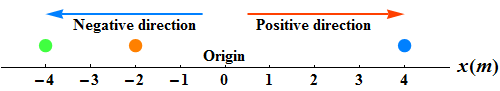The positive direction of the axis is in the direction of increasing numbers, which is in the right

The opposite is the negative direction.

Position is a vector quantity as it has direction (+ or – in one dimension) and magnitude.

What is the position of Green, Orange and Blue disks?

Green is -4 m, Red is -2 m and Blue is +4 m.

Displacement

What is the position of red disc?

Move (click play) it to a new position.

Displacement is the change from one position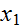to another position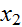.

Displacement is a vector quantity with direction (+ or – in one dimension) and magnitude.

How displacement is computed?

Displacement is computed by subtracting initial positionfrom final position.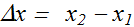Symbol Δ (uppercase delta), represents a change in quantity (here it is change in position)

What will be displacement if object moves fromto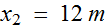?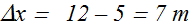What is the direction of motion?

Positive sign of Δx indicates that the motion is along the positive x-direction

What will be displacement if the object moves from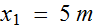to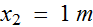?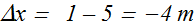What is the direction of motion?

Negative sign of Δx indicates that the motion is along the negative x-direction

Displacement vs. Total Distance

Watch the motion of red disk below (click play).

Blue arrow is the instantaneous displacement.

The disk moved from initial position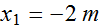to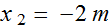.  Even though the total distance (dotted line) covered is 10 m the displacement  is still  Δx = 0.Now watch the motion of blue disk (click play) below.

Red arrow is the displacement.

The disk moved from initial positionto.  Even though the total distance (dotted line) covered is 8 m the displacement  is  Δx = 2m.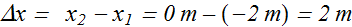The actual distance for a trip is irrelevant as far as the displacement is concerned

Position time Graph

Position of a moving object (blue disk) can be plotted as a function of time.

Note down position of moving disk (blue) for different values of time t.

These various noted values of points (t, x) can be plotted as a graph with position x(t) at a given time t on Vertical axes and time t on horizontal axes.

This is called time versus position graph or position time graph.

Stationary Object

When the position of an object or a particle does not change with time, it is called a stationary object or particle.

What we observed?

In other words displacement of the particle is zero with time.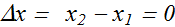Position time graph of a stationary object is parallel to time axes.

The position of the object stays at x=-5 m for 10 s.

Average Velocity

Average velocity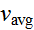, is the ratio of the displacement Δx that occurs during a particular time interval Δt to that interval.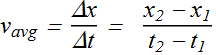On a position x versus t graph,is the slope of the straight line (Magenta line) that connects two particular points on the x (t) curve : one point is (,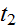), and other point is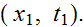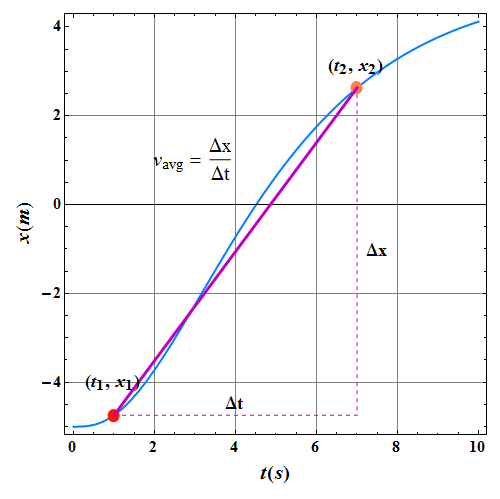Average Speed

Average speed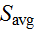, is the ratio of the total distance covered during a particular time interval Δt to that interval.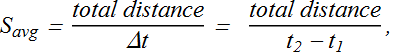Average speed does not include direction, it lacks any algebraic sign.

Some timeis the same (except for the absence of sign) as. As in the following example.

In the above example Δx = 5 m and total distance covered is also 5 m.

However (following example) when an object double back on its path, the two can be quite different.

In the above example Δx = 2 m (red arrow) while total distance covered is 8 m.

Instantaneous Velocity and Speed

Instantaneous velocity v is the change in particle position x with time at a given instant.

How to compute instantaneous velocity?

First calculate average velocity around the given instant (red circle).

Now shrink the time interval Δt (using Δt slider) closer and closer to 0.

As Δt becomes shorter and shorter the average velocity approaches a limiting value, which is the instantaneous velocity (or simply velocity).

Mathematically v is the derivative of x with respect to t.In graphical term, velocity v at given instant is the slope of the particle’ s position - time curve at the point representing that instant.

Instantaneous speed (Speed) is the magnitude of velocity.

Time vs. Velocity Graph

We are given a position time graph, how can we obtain velocity time graph from this?

We can measure slope of the curve at each time position (red circle).

Value of slope is velocity v at that instant (time).

These various noted values of points (t, v) can be plotted as a graph with velocity v(t) on Vertical axes and time t on horizontal axes.

Acceleration

When a particle's velocity changes, the particle is said to undergo acceleration (or to accelerate).

For motion along an axis, the average acceleration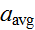over a time interval Δt is defined as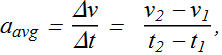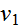is the particle velocity at time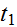andis particle velocity at.

The instantaneous acceleration (or simply acceleration) is the derivative of the velocity with respect to time: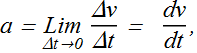Acceleration of a particle at any instant is the rate at which its velocity is changing at that instant.

Units of Acceleration

We found that acceleration is derivative of velocity with time.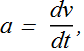Now velocity is derivative of position x(t) with time, therefore at any instant acceleration is the second derivative of its position x(t) with respect to time.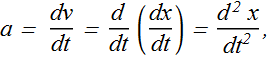Unit of acceleration is the m/(s.s) or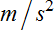.

Information from sign of Acceleration

Positive sign of acceleration indicates it is acting to the right and negative sign indicates it is acting to the left.

Just form the sign of acceleration, can we tell whether an object's speed is increasing or decreasing?

We need to know sign of velocity too.

If the signs of the velocity and acceleration of a particle are the same, the speed of the particle increases. If the signs are opposite, the speed decreases.

Time vs. Acceleration Graph

We have a velocity time graph, how can we obtain acceleration time graph from this?

We can measure slope of the curve at each time position (red circle).

Value of slope is acceleration a at that instant (time).

These various noted values of points (t, a) can be plotted as a graph with acceleration a(t) on Vertical axes and time t on horizontal axes.

Constant Acceleration

Let us explore how various graphs look like for different values of velocity and acceleration.

When acceleration is constant, how the slope of velocity time graph change with time?

It is constant.

Equations of Motion (Kinematic Equations)

When the acceleration is constant, the average acceleration is equal to the instantaneous acceleration.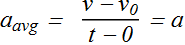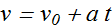Here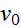is the velocity at time t=0 and v is the velocity at any later time t.

For constant acceleration the average velocityover a time interval t can also be given as the average of the initial velocityand final velocity v.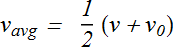When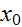is the initial position and x is the position at time t, then average velocityis given as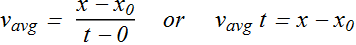Combining above equations we get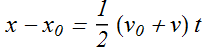Kinematic Equations

For Constant acceleration, with the help of above two equations we can find following list of equations of motion.

 Equation Quantity Missing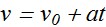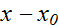v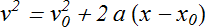t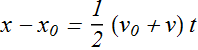a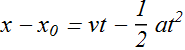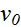All these equations are only valid for constant acceleration.

Free Fall Acceleration

Free fall acceleration is an example of constant acceleration.

When we toss an object either up or down and could somehow eliminate the effects of air on its flight, we would find that the object accelerates downward at a constant rate.

That rate is called the free-fall acceleration, and its magnitude is represented by g.

The free-fall acceleration is negative: that is, downward on the y axis, toward Earth's center.

The free-fall acceleration near Earth's surface is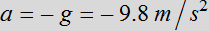, and the magnitude of the acceleration is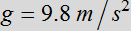. Do not substitute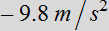for g.

Graphical Integration in Motion Analysis

Can we calculate the displacement, if we know time versus velocity graph representing motion of a particle?

Yes, we can compute the displacement between any time interval (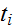to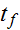)

Velocity v is defined as derivative of position with time.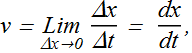In other words for a very small interval of time (Δt→0), the displacement Δx can be given as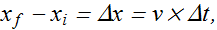Example 1:

Suppose the time versus velocity plot of a moving object is as follow.

If we want to compute displacement from initial time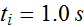to final time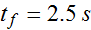, we can divide this time into four equal parts of  Δt=0.5s each (Select Show).

Now total displacement will be the sum of displacement in these four small intervals.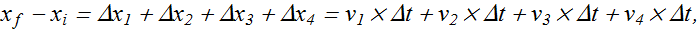To increase the accuracy of our calculations, we need to make Δt as small as possible, therefore the displacement will be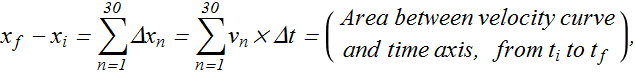For further accuracy we can make Δt very small (Δt→0) and Fundamental Theorem of Calculus tells us that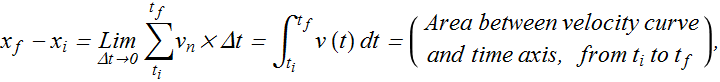Example 2 :

When we have a graph of an object's time versus acceleration, we can integrate on the graph to find the object's velocity at any given time (If initial or final velocity is also known).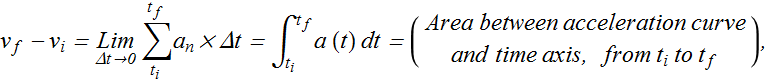Here plot of time versus acceleration of a moving object (acceleration is not constant) is given.

Graphical methods are valid, whether acceleration is constant or not.